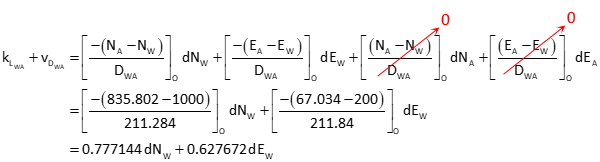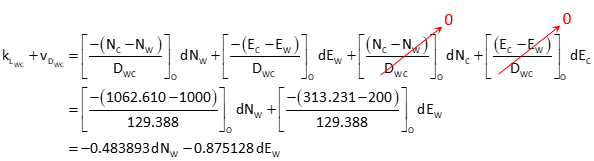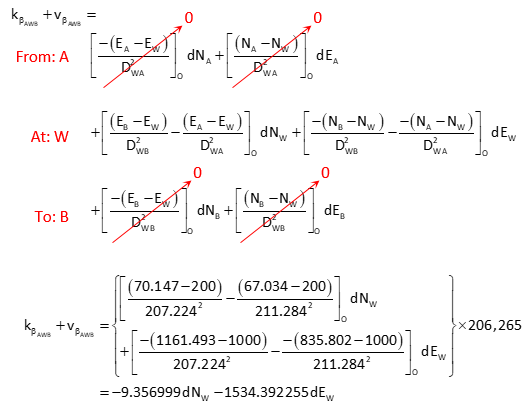### Problem (3)

Given the horizontal network below:The initial coordinates of point W are estimated.

Create the [C] and [K] matrices.

#### Solution

The coordinates are large and manipulating them could lead to potential rounding errors. To simplify computations a bit, the coordinates can be reduced to a local origin. This is done by subtracting one constant from the E coordinates and another from the N. In this case, subtract 42,600 m from E coordinates and 115,000 m from N:

 Point E N A 67.034 835.802 B 70.147 1161.493 C 313.231 1062.610 W 200 1000

Matrices structuresDistances and angles from initial approximations[K] matrix elementsCompute [C] matrix elements

Distance WADistance WCAngle AWBAngle BWCAssemble [C] and [K] matrices.